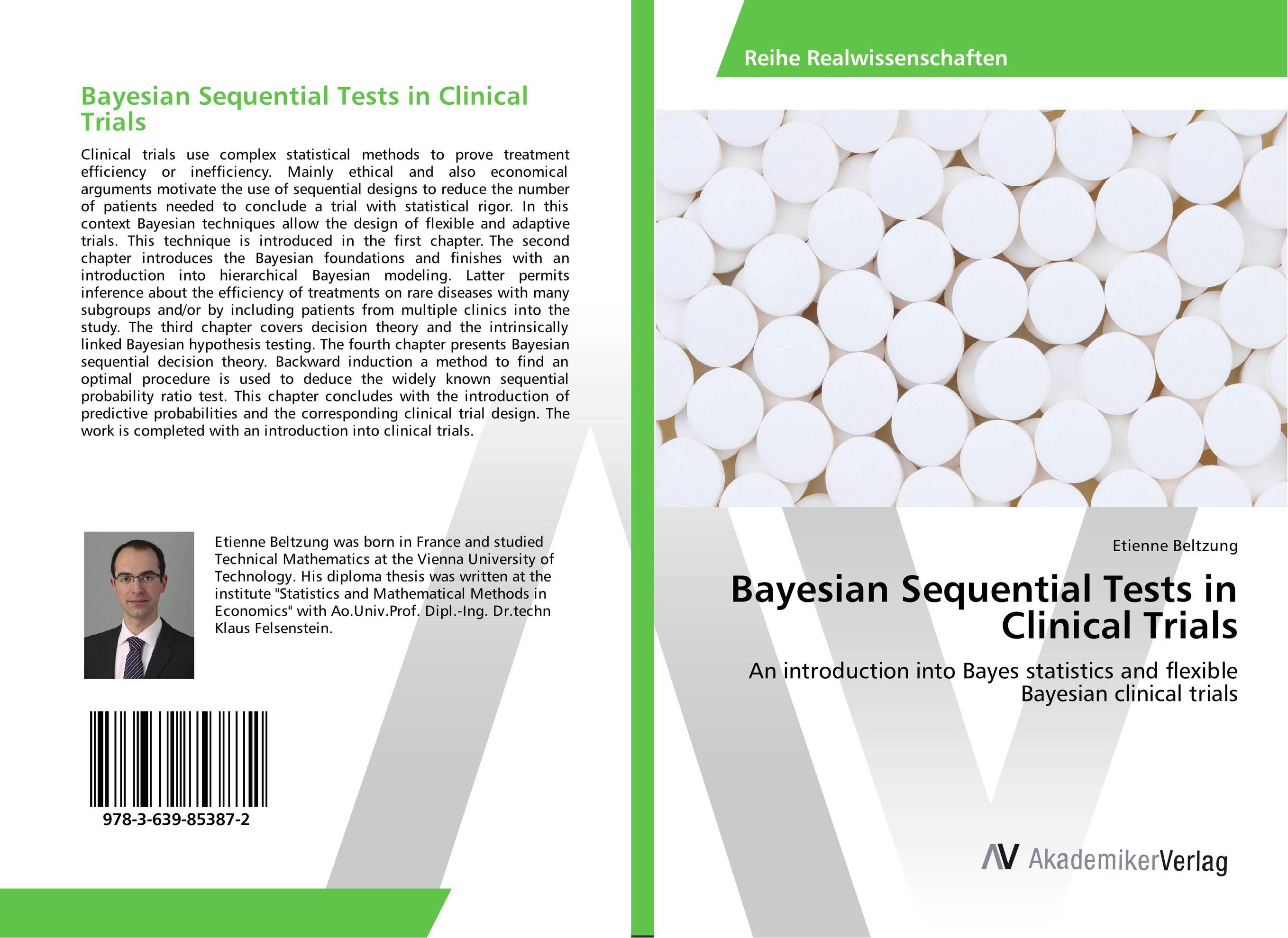# Bayesian Sequential Tests in Clinical Trials

###### Аннотация:
Clinical trials use complex statistical methods to prove treatment efficiency or inefficiency. Mainly ethical and also economical arguments motivate the use of sequential designs to reduce the number of patients needed to conclude a trial with statistical rigor. In this context Bayesian techniques allow the design of flexible and adaptive trials. This technique is introduced in the first chapter. The second chapter introduces the Bayesian foundations and finishes with an introduction into hierarchical Bayesian modeling. Latter permits inference about the efficiency of treatments on rare diseases with many subgroups and/or by including patients from multiple clinics into the study. The third chapter covers decision theory and the intrinsically linked Bayesian hypothesis testing. The fourth chapter presents Bayesian sequential decision theory. Backward induction a method to find an optimal procedure is used to deduce the widely known sequential probability ratio test. This chapter concludes with the introduction of predictive probabilities and the corresponding clinical trial design. The work is completed with an introduction into clinical trials.
###### Издания произведения:9783639853872

### Рекомендуем

#### Комментарии

Пока нет ни одного комментария
Вы должны войти для того что бы оставлять комментарии